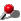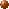# Representation of Program Text

Program text is accessible at the meta level in the form of parse tree. The parse tree is represented by a `Ptree` metaobject. It is implemented as a nested linked-list of lexical tokens --- the S expressions in the Lisp terminology. For example, this piece of code:

``````int a = b + c * 2;
``````

is parsed into:

``````[[static] [int] [[a = [b + [c * 2]]]] ;]
``````

Here, `[]` denotes a linked list. Note that operators such as `=` and `+` make sublists. The sublists and their elements (that is, lexical tokens such as `a` and `=`) are also represented by `Ptree` metaobjects.

##Public Members

```Append(Ptree* lst1, Ptree* lst2)Cons(Ptree* a, Ptree* b)CopyList(Ptree* lst)Display()Eq(Ptree* lst, char x)Eq(Ptree* lst, char* x)Eq(Ptree* lst, Ptree* x)Equal(Ptree* x, Ptree* y)First(Ptree* lst)GenSym()IsLeaf()Last(Ptree* lst)Length(Ptree* lst)List(Ptree* e1, Ptree* e2, ...)ListTail(Ptree* lst, int k)Make(char* format, [Ptree* sublist, ...])Match(Ptree* list, char* pattern, [Ptree** sublist, ...])Nth(Ptree* lst, int n)Reify(unsigned int& value)Reify(char*& string)ReplaceAll(Ptree* lst, Ptree* orig, Ptree* subst)operator <<(ostream& s, Ptree* p)Rest(Ptree* lst)Second(Ptree* lst)Third(Ptree* lst)ToString()Write(ostream& out)qMake(char* text) ```

##Basic Operations

To manipulate linked lists, the MOP provides many `static` member functions on `Ptree`, which are familiar to Lisp programmers:

```static Ptree* First(Ptree* lst)
```

This returns the first element of `lst`.

```static Ptree* Rest(Ptree* lst)
```

This returns the rest of `lst` except the first element, that is, the cdr field of `lst`.

```static Ptree* Second(Ptree* lst)
```

This returns the second element of lst.

```static Ptree* Third(Ptree* lst)
```

This returns the third element of lst.

```static Ptree* Nth(Ptree* lst, int n)
```

This returns the `n`-th element of `lst`. `Nth(lst, 0)` is equivalent to `First(lst)`.

```static Ptree* Last(Ptree* lst)
```

This returns the last cons cell, which is a list containing only the last element of `lst`.

```static Ptree* ListTail(Ptree* lst, int k)
```

This returns a sublist of `lst` obtained by omitting the first `k` elements. `ListTail(lst, 1)` is equivalent to `Rest(lst)` .

```static int Length(Ptree* lst)
```

This returns the number of the elements of `lst`. If `lst` is not a list, then this returns a negative number.

```static Ptree* Cons(Ptree* a, Ptree* b)
```

This returns a cons cell whose car field is `a` and whose cdr is `b`.

```static Ptree* List(Ptree* e1, Ptree* e2, ...)
```

This returns a list whose elements are `e1`, `e2`, ... `List()` returns a null list `nil`.

```static Ptree* Append(Ptree* lst1, Ptree* lst2)
```

This concatenates `lst1` and `lst2`. It returns the resulting list.

```static Ptree* CopyList(Ptree* lst)
```

This returns a new list whose elements are the same as `lst` 's.

```static Ptree* ReplaceAll(Ptree* lst, Ptree* orig, Ptree* subst)
```

This returns a list in which all occurrences of `orig` in `lst` are replaced with `subst`. This is not a destructive operation.

```static bool Eq(Ptree* lst, char x)static bool Eq(Ptree* lst, char* x)static bool Eq(Ptree* lst, Ptree* x)
```

This returns `true` if `lst` and `x` are equal. If `x` is `Ptree*`, this determines the equivalence by comparing the pointers.

```static bool Equal(Ptree* x, Ptree* y)
```

This recursively compares `x` and `y` and returns `true` if they are equivalent.

Furthermore, the following member functions are available on `Ptree` metaobjects:

`bool IsLeaf()`

This returns `true` if the metaobject indicates a lexical token.

`void Display()`

This prints the metaobject on the console for debugging. Sublists are surrounded by `[` and `]`.

`char* ToString()`

This converts the parse tree into a character string and returns it.

`int Write(ostream& out)`

This writes the metaobject to the file specified by `out`. Unlike `Display()` , sublists are not surrounded by `[` and `]`. This member function returns the number of written lines.

`ostream& operator <<(ostream& s, Ptree* p)`

The operator `<<` can be used to write a `Ptree` object to an output stream. It is equivalent to `Write()` in terms of the result.

The parse tree is basically a long list of the lexical tokens that appear in the program although some of them are grouped into sublists. The order of the elements of that list is the same as the order in which the lexical tokens appear. But if some fields such as the type field are omitted in the program, then `nil` is inserted at those places. For example, if the return type of a function declaration is omitted as follows:

``````main(int argc, char** argv){ }
``````

then `nil` list is inserted at the head of the list:

``````[nil nil [main ( [[[int] [argc]] , [[char] [* * argv]]] )] [{
nil
}]
``````

Since the function body is also omitted, `nil` list is inserted between `{` and `}`.

##Construction

Programmers can make `Ptree` metaobjects. Because the MOP provides a conservative garbage collector, they don't need to care about deallocation of the metaobjects. The next `static` member functions on `Ptree` are used to make a `Ptree` metaobjects.

```static Ptree* Make(char* format, [Ptree* sublist, ...])
```

This makes a `Ptree` metaobject according to the `format`. The `format` is a null-terminated string. All occurrences of `%c` (character), `%d` (integer), `%s` (character string), and `%p` (`Ptree`) in the `format` are replaced with the values following the `format`. `%%` in the `format` is replaced with `%`.

```static Ptree* GenSym()
```

This generates a unique symbol name (aka identifier) and returns it. The returned symbol name is used as the name of a temporary variable, for example.

The `Ptree` metaobject returned by `Make()` is not a real parse tree.(At least, for the time being.)It is just a unparsed chunk of characters. Although programmers can use `Ptree` metaobjects generated by `Make()` as they use other `Ptree` metaobjects, the structure of those metaobjects does not reflect the code they represent.

Using `Make()`, programmers can easily generate any piece of code to substitute for part of the original source code. For example, suppose `array_name` is `xpos` and `offset` is `3`. The following function call:

``````Ptree::Make("%p[%d]", array_name, offset)
``````

makes a `Ptree` metaobject that represents:

``````xpos
``````

`%p` simply expand a given `Ptree` metaobject as a character string. Thus programmers may write something like:

``````Ptree::Make("char* GetName(){ return \"%p\"; }",
array_name);
``````

Note that a double quote `"` must be escaped by a backslash `\` in a C++ string. `\"%p\"` makes a string literal. The function call above generates the code below:

``````char* GetName(){ return "xpos"; }
``````

Although `Make()` follows the old `printf()` style, programmers can also use a more convenient style similar to Lisp's backquote notation. For example,

``````Ptree::Make("%p[%d]", array_name, offset)
``````

The expression above can be rewritten using `qMake()` as follows:

``````Ptree::qMake("`array_name`[`offset`]")
``````

Note that the ``backqouted'' C++ expressions `array_name` and `offset` are directly embedded in the C++ string. Their occurrence are replaced with the value of the expression. This replacement cannot be implemented in regular C++. It is implemented by the metaclass for `Ptree`.

```static Ptree* qMake(char* text)
```

This makes a `Ptree` metaobject that represents the `text`. Any C++ expression surrounded by backquotes ``` can appear in `text`. Its occurrence is replaced with the value denoted by the expression. The type of the expression must be `Ptree*`, `int`, or `char*`.

Except the difference in the notation, `qMake()` is equivalent to `Make()`. Programmers can choose either one they prefer at any place.

##Pattern Matching

The MOP provides a `static` member function on `Ptree` metaobjects for pattern matching.

```static BOOL Match(Ptree* list, char* pattern,
[Ptree** sublist, ...])
```

This compares the `pattern` and `list`. If they match, this function returns `true` and binds the `sublist`s to appropriate sublists of the `list`, as specified by the `pattern`. Note that the type of `sublist` is pointer to `Ptree*`.

For example, the function `Match()` is used as follows:

``````if(Ptree::Match(expr, "[%? + %?]", &lexpr, &rexpr))
cout << "this is an addition.";
else if(Ptree::Match(expr, "[%? - %?]", &lexpr, &rexpr))
cout << "this is a subtraction.";
else
cout << "unknown";
``````

The pattern `[%? + %?]` matches a linked list that consists of three elements if the second one is `+`. If an expression `expr` matches the pattern, `lexpr` gets bound to the first element of `expr` and `rexpr` gets bound to the third element.

The `pattern` is a null-terminated string. Since `Match()` does not understand the C++ grammar, lexical tokens appearing in the pattern must be separated by a white space. For example, a pattern `a+b` is regarded as a single token. The pattern is constructed by these rules:

1. A word (characters terminated by a white space) is a pattern that matches a lexical token.
2. `%[`, `%]`, and `%%` are patterns that match `[`, `]`, and `%`.
3. `[]` is a pattern that matches a null list (`nil`).
4. `[`pat1 pat2 ... `]` is a pattern that matches a list of pat1, pat2, ...
5. `%*` is a pattern that matches any token or list.
6. `%?` is a pattern that matches any token or list. The matched token or list is bound to `sublist`.
7. `%_` is a pattern that matches the rest of the list (the `cdr` part).
8. `%r` is a pattern that matches the rest of the list. The matched list is bound to `sublist`.

##Reifying Program Text

If a `Ptree` metaobject represents a literal such as an integer constant and a string literal, we can obtain the value denoted by the literal.

```static BOOL Reify(unsigned int& value)
```

This returns `true` if the metaobject represents an integer constant. The denoted value is stored in `value`. Note that the denoted value is always a positive number because a negative number such as `-4` generates two distinct tokens such as `-` and `4`.

```static BOOL Reify(char*& string)
```

This returns `true` if the metaobject represents a string literal. A string literal is a sequence of character surrounded by double quotes `"`. The denoted null-terminated string is stored in `string`. It does not include the double quotes at the both ends. Also, the escape sequences are not expanded.

Note: the character string returned by `Reify()` is allocated in the heap area. However, because the MOP provides a conservative garbage collector, programmers do not need to deallocate the string by themselves.

##Support Classes

The MOP provides two support classes `PtreeIter` and `PtreeArray` to help programmers to deal with `Ptree` objects. `PtreeIter` is useful to perform iteration on a list of `Ptree` objects. Suppose that `expr` is a list:

``````PtreeIter next(expr);
Ptree* p;
while((p = next()) != nil){
// compute on p
}
``````

Each element of `expr` is bound to `p` one at a time. The operator `()` on `PtreeIter` objects returns the next element. Programmers may call `Pop()` instead of the operator `()`. Since the two functions are equivalent, the program above can be rewritten to be:

``````PtreeIter next(expr);
Ptree* p;
while((p = next.Pop()) != nil){
// compute on p
}
``````

If the reader prefers the `for`-loop style, she may also say:

``````for(PtreeIter i = expr; !i.Empty(); i++){
// compute on *i
}
``````

Although this interface is slightly slower, it distinguishes the end of the list and a `nil` element. If `expr` includes `nil`, `Pop()` cannot correctly detect the end of the list.

Another support class is `PtreeArray` for dealing with an unbounded array of `Ptree` objects. It is used as follows (suppose that `expr` is a `Ptree` object):

``````PtreeArray a;            // allocate an array
a.Append(expr);          // append expr to the end of the array
Ptree* p = a;         // get the first element
Ptree* p2 = a.Ref(0);    // same as a
int n = a.Number();      // get the number of elements
Ptree* lst = a.All();    // get a list of all the elements
a.Clear();               // make the array empty
``````

[First | Prev | Next]